Courses

# Test: Dienes- Hydrocarbons

## 10 Questions MCQ Test Chemistry Class 11 | Test: Dienes- Hydrocarbons

Description
This mock test of Test: Dienes- Hydrocarbons for JEE helps you for every JEE entrance exam. This contains 10 Multiple Choice Questions for JEE Test: Dienes- Hydrocarbons (mcq) to study with solutions a complete question bank. The solved questions answers in this Test: Dienes- Hydrocarbons quiz give you a good mix of easy questions and tough questions. JEE students definitely take this Test: Dienes- Hydrocarbons exercise for a better result in the exam. You can find other Test: Dienes- Hydrocarbons extra questions, long questions & short questions for JEE on EduRev as well by searching above.
QUESTION: 1

### Direction (Q. Nos. 1 - 6) This section contains 6 multiple choice questions. Each question has fo ur choices (a), (b), (c) and (d), out of which ONLY ONE option is correct. Q. The correct increasing order of heat evolved when 1.0 mole of each of the following is hydrogenated completely is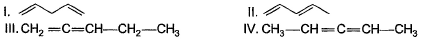Solution:

In the case of alkene, we have the order of stability as “ resonance>isolated>continuous pi bond”. The reason behind this is due to the presence of continuous Pi bond there is repulsion between the cloud of electrons and thus the alkene molecules with continuous pi bond become unstable. The heat of hydrogenation order is reverse the order of stability. We will find the stability. Due to resonance second is more stable than first. In three and four four is more stable as it has 6 Alpha hydrogen while 3  has only two Alpha hydrogen so the order of stability is  2>1>4>3, so the heat of hydrogenation order will be the reverse of this and the Order will be option number d

QUESTION: 2

### Consider the following transformations.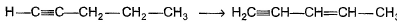Q. Which reaction sequence will bent bring about the above transformation?

Solution:On addition of H2/Pd/CaCO3
we get H-CH=C-CH2-CH2-CH3
H-CH=C-CH2-CH2-CH3 + NBS/CCl4   →   H-CH=C-CHCl-CH2-CH3  (NBS adds Br to carbon at next alternate to double bond)
H-CH=C-CHCl-CH2-CH3 + KOH/EtOH  →  H2C=C-CH=CH-CH3
(by E2 elimination)

QUESTION: 3

### What is the major formed in the reaction?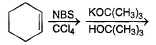Solution:

For option a
12 = [Ne]3s2 , 20 = [Ar]4s2 , 4 = [He]2s2 , 88 = [Ra]7s2.
For option b
8 = [He]2s22p4 , 16 = [Ne]3s23p4 , 34 = [Ar]4s23d104p4 , 2 = 1s2
We can see that only in option a, the last electrons enter in similar group. So, option a is correct.
Similarly we can check for option c and d as done above. However they won't fall in the same group.

QUESTION: 4

What is the major product formed in the reaction below?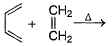Solution:

The correct answer is option D
Diels - Alder reaction: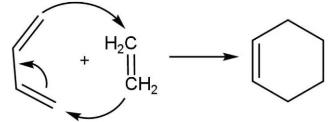QUESTION: 5

What is formed in the reaction below as major product?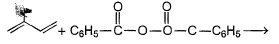Solution:

The correct answer is Option C.
A plane of symmetry is an imaginary plane that bisects a molecule into halves that are mirror images of each other.
A molecule has a center of symmetry when, for any atom in the molecule, an identical atom exists diametrically opposite this center an equal distance from it. In other words, a molecule has a center of symmetry when the points (x,y,z) and (−x,−y,−z) correspond to identical objects. For example, if there is an oxygen atom in some point (x,y,z), then there is an oxygen atom in the point (−x,−y,−z). There may or may not be an atom at the inversion center itself. Examples are xenon tetrafluoride where the inversion center is at the Xe atom, and benzene (C6H6) where the inversion center is at the center of the ring.
Optical activity is the ability of a chiral molecule to rotate the plane of plane-polarised light.

QUESTION: 6

What is the major product in the reaction below?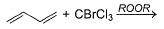Solution:
QUESTION: 7

Direction (Q. Nos. 7 - 10) This section contains 4 multiple choice questions. Each question has four choices (a), (b), (c) and (d), out of which ONE or MORE THAN ONE are correct.

Q. Predict product(s) in the following reaction.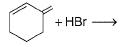Solution:

In Benzene ring, the meta position is more stable than ortho and para.
At high temperature meta position will exist
i.e option B is correct

*Multiple options can be correct
QUESTION: 8

The correct statement(s) regarding following reaction is/are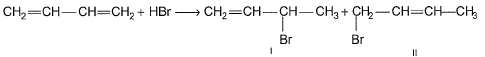Solution:
QUESTION: 9

Predict product(s) of the following reactions.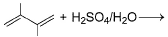Solution:

The correct answer is Option D.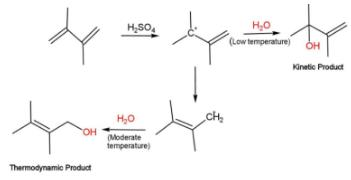*Multiple options can be correct
QUESTION: 10